## Free self marking computing tests for binary, decimal and hex conversion

Converting from binary to decimal and back again is a key skill for Computer Scientists at GCSE and above. Students also have to be able to convert to and from hexadecimal.

This means that there are 6 possible conversions that students need to be able to make in a Computer Science exam: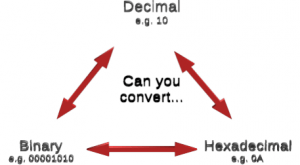Can you convert between binary, hex and decimal

I’ve updated this free tool so that you can generate 20 questions to test yourself (or your students) on any of the six conversions above. The self marking computing tests let you choose which conversions you want to practice and will give you an accuracy and speed score as well as a final score for the number you manage to get correct.

Click here to try out the self marking tests.

## Free binary teaching tool: How does 2s complement work?

My GCSE Computer Science students need to understand how to convert positive numbers into 8 bit binary. Today, my Y10s had to teach this to the Y6s visiting for their new Y7 induction day. After the  Y6s had gone off for an early lunch, my Y10s had to discuss how they would extend what they knew about binary to allow computers to understand negative numbers as well as positive numbers.

The end result was an explanation of 2’s complement and how it works, using this free interactive binary teaching tool.

What is binary?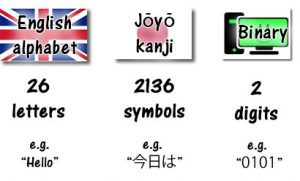Binary is made up of 0s and 1s

There are 26 letters in the English alphabet. Even in simplified Japanese Kanji, there are over 2,000 symbols. The digital electronics that makes computers work can only understand two possible states: electricity switched on and electricity switched off. That’s why binary is used so much in computers: a binary 1 or 0 can be processed with electronic switches and stored in electronic ‘memory’.

Even though computers only ‘understand’ 0s and 1s, they can combine multiple 0s and 1s to represent any number, image, sound or anything else.Free teaching aid: How does 2s complement work?

How can positive numbers be represented in binary?

In the same way that you can combine letters in the English alphabet to make any word, you can combine 0s and 1s in binary to represent any sort of data.

The easiest sort of data to represent is an unsigned integer.

Unsigned means that it’s a positive number: it has no + or – sign.

Integer means that it’s a whole number: it has no decimal point.

Each individual 0 or 1 in a binary number is called a bit. As you look from right to left at a binary number, each bit is worth twice as much as the one on its right.

Example: 01001001

The bit on the far right is known as the least significant bit: it’s only worth 1.

As you move from right to left, each bit doubles in value, until you get to the bit on the far left, which we call the most significant bit: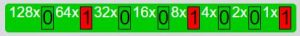01001001

To convert from binary into decimal (what most people think of as ‘normal’ numbers) you have to add together all of the values of bits that are set to 1.

For our example of 01001001, you would add together 64 + 8 + 1 = 73

Experiment with this interactive tool to see how to convert from binary to decimal and vice versa.

How can negative numbers be represented in binary?

When asked this question, my Y10s came up with a couple of suggestions:

• You could use twice as many bits to store each number: each bit could have an extra ‘positive or negative’ bit next to it

This would work but would be a massive waste of memory: you’d need twice as much RAM to store negative numbers and you’d have to redesign CPUs to handle negative numbers in a different way to positive numbers. Converting between the two types would be a waste of processing power.

• You could add an extra bit on to the end you binary number: just one ‘positive or negative’ bit at the start of each number

This is a much better idea but you’d still be wasting memory: an 8 bit number would need 9 bits in total. A 32 bit number would need 33 bits etc… That extra bit will cause problems when you try to store it as almost all memory is designed for multiples of 8 bits: 8, 16, 32, 64, 128 bit etc…

So, the way forwards is to take the most significant bit of a binary number (the bit on the far left) and keep the same absolute value but make it negative.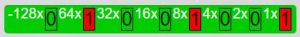8 bit 2s complement binary bit values

Note that the most significant bit (MSB) is now worth -128 rather than 128.

The advantage of representing negative numbers this way is that for any positive number where the MSB was 0, the binary is the same as it would be if we’d used our good old fashioned normal way of representing binary numbers. You can also quickly tell if any number is negative by looking at the MSB: it’s negative if the MSB is 1 and it’s positive (or zero) if the MSB is 0.

This method of representing negative numbers in binary is called 2’s complement.

Experiment with this interactive tool to see how to convert from binary to decimal and vice versa. Tick the 2s complement box at the top to experiment with negative numbers.

How do you convert a 2’s complement binary number into decimal?

This is easy. You’d do exactly the same as you would for a positive number: give the least significant bit (on the right) a value of 1 and double the value of each bit as you move to the left. Add up all the values of bits that are 1s. The only difference is that the most significant bit (on the left) is negative:

Example: 10001000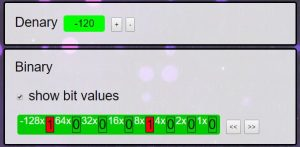10001000 in 2s complement binary

In this example, the MSB (most significant bit, on the left) is set to 1 so we can know that the number is negative.

When you add together the values of each bit that is set to 1 you get:

-128 + 8 = -120

So the 2s complement binary number 10001000 represents the negative number -120.

The digital electronics that makes a computer work doesn’t know (or care) if 10001000 is 2s complement or not. It could just as easily represent the positive number 136 (128 + 8). The computer processes the binary data (for addition, subtraction etc…) in exactly the same way. It’s down to the software rather than the hardware to remember if the binary data is a signed (2s complement) number or an unsigned (positive) number. This can sometimes lead to some pretty catastrophic software failures when programmers aren’t aware of how computers handle negative numbers.

Experiment with this interactive tool to see how to convert from binary to decimal and vice versa. Tick the 2s complement box at the top to experiment with negative numbers.